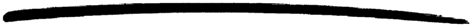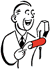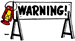## 4 Tables and databases

### 4.1 Ets, Dets and Mnesia

All examples using Ets has an corresponding example in Mnesia. In general all Ets examples also applies to Dets tabels.

#### 4.1.1 Select/Match operations

Select/Match operations on Ets and Mnesia tables can become very expensive operations. They will have to scan the whole table that may be very large. You should try to structure your data so that you minimize the need for select/match operations. However if you need a select/match operation this will be more efficient than traversing the whole table using other means such as `tab2list` and `mnemosyne`. Examples of this and also of ways to avoid select/match will be provided in some of the following sections. From R8 the functions `ets:select/2` and `mnesia:select/3` should be preferred over `ets:match/2`,`ets:match_object/2` and `mnesia:match_object/3`.There are exceptions when the whole table is not scanned. This is when the key part is bound, the key part is partially bound in an `orded_set` table, or if it is a mnesia table and there is a secondary index on the field that is selected/matched. Of course if the key is fully bound there will be no point in doing a select/match, unless you have a bag table and you are only interested in a sub-set of the elements with the specific key.

When creating a record to be used in a select/match operation you want most of the fields to have the value '_'. To avoid having to explicitly set all of these fields people have created functions that takes advantage of the fact that records are implemented as tuples. This is not such a good idea, that is why you from R8 can do the following

```#person{age = 42, _ = '_'}.

```

This will set the age attribute to 42 and all other attributes to '_'. This is more efficient then creating a tuple with '_' values, that then is used as a record. It is also much better code as it does not violate the record abstraction.

#### 4.1.2 Deleting an element

As in the case of lists, the delete operation is considered successful if the element was not present in the table. Hence all attempts to check that the element is present in the Ets/Mnesia table before deletion are unnecessary. Here follows an example for Ets tables.

DO

```...
ets:delete(Tab, Key),
...

```

DO NOT

```...
case ets:lookup(Tab, Key) of
[] ->
ok;
[_|_] ->
ets:delete(Tab, Key)
end,
...

```

#### 4.1.3 Data fetching

Do not fetch data that you already have! Consider that you have a module that handles the abstract data type Person. You export the interface function `print_person/1` that uses the internal functions `print_name/1`, `print_age/1`, `print_occupation/1`.If the functions `print_name/1` etc. had been interface functions the matter comes in to a whole new light. As you do not want the user of the interface to know about the internal data representation.

DO

```%%% Interface function
print_person(PersonId) ->
%% Look up the person in the named table person,
case ets:lookup(person, PersonId) of
[Person] ->
print_name(Person),
print_age(Person),
print_occupation(Person);
[] ->
io:format("No person with ID = ~p~n", [PersonID])
end.

%%% Interanal functions
print_name(Person) ->
io:format("No person ~p~n", [Person#person.name]).

print_age(Person) ->
io:format("No person ~p~n", [Person#person.age]).

print_occupation(Person) ->
io:format("No person ~p~n", [Person#person.occupation]).

```

DO NOT

```%%% Interface function
print_person(PersonId) ->
%% Look up the person in the named table person,
case ets:lookup(person, PersonId) of
[Person] ->
print_name(PersonID),
print_age(PersonID),
print_occupation(PersonID);
[] ->
io:format("No person with ID = ~p~n", [PersonID])
end.

%%% Interanal functions
print_name(PersonID) ->
[Person] = ets:lookup(person, PersonId),
io:format("No person ~p~n", [Person#person.name]).

print_age(PersonID) ->
[Person] = ets:lookup(person, PersonId),
io:format("No person ~p~n", [Person#person.age]).

print_occupation(PersonID) ->
[Person] = ets:lookup(person, PersonId),
io:format("No person ~p~n", [Person#person.occupation]).

```

#### 4.1.4 Non persistent data storage

For non persistent database storage, prefer Ets tables before Mnesia local_content tables. Even the cheapest Mnesia operations, `dirty_write` operations, carry a fixed overhead compared to Ets writes. Mnesia must check if the table is replicated or has indices, this involves at least one Ets lookup for each `dirty_write`. Thus, Ets writes will always be faster than Mnesia writes.

#### 4.1.5 tab2list

Assume we have an Ets-table, which uses `idno` as key, and contains:

```[#person{idno = 1, name = "Adam",  age = 31, occupation = "mailman"},
#person{idno = 2, name = "Bryan", age = 31, occupation = "cashier"},
#person{idno = 3, name = "Bryan", age = 35, occupation = "banker"},
#person{idno = 4, name = "Carl",  age = 25, occupation = "mailman"}]

```

If we must return all data stored in the Ets-table we can use `ets:tab2list/1`. However, usually we are only interested in a subset of the information in which case `ets:tab2list/1` is expensive. If we only want to extract one field from each record, e.g., the age of every person, we should use:

DO

```...
ets:select(Tab,[{ #person{idno='_',
name='_',
age='\$1',
occupation = '_'},
[],
['\$1']}]),
...

```

DO NOT

```...
TabList = ets:tab2list(Tab),
lists:map(fun(X) -> X#person.age end, TabList),
...

```

If we are only interested in the age of all persons named Bryan, we should:

DO

```...
ets:select(Tab,[{ #person{idno='_',
name="Bryan",
age='\$1',
occupation = '_'},
[],
['\$1']}]),
...

```

DO NOT

```...
TabList = ets:tab2list(Tab),
lists:foldl(fun(X, Acc) -> case X#person.name of
"Bryan" ->
[X#person.age|Acc];
_ ->
Acc
end
end, [], TabList),
...

```

REALLY DO NOT

```...
TabList = ets:tab2list(Tab),
BryanList = lists:filter(fun(X) -> X#person.name == "Bryan" end,
TabList),
lists:map(fun(X) -> X#person.age end, BryanList),
...

```

If we need all information stored in the ets table about persons named Bryan we should:

DO

```...
ets:select(Tab, [{#person{idno='_',
name="Bryan",
age='_',
occupation = '_'}, [], ['\$_']}]),
...

```

DO NOT

```...
TabList = ets:tab2list(Tab),
lists:filter(fun(X) -> X#person.name == "Bryan" end, TabList),
...

```

#### 4.1.6 Ordered_set tables

If the data in the table should be accessed so that the order of the keys in the table is significant, the table type `ordered_set` could be used instead of the more usual `set` table type. An `ordered_set` is always traversed in Erlang term order with regards to the key field so that return values from functions such as `select `, `match_object` and `foldl` are ordered by the key values. Traversing an `ordered_set` with the first and next operations also returns the keys ordered.An `ordered_set` only guarantees that objects are processed in key order. Results from functions as `ets:select/2` appear in the key order even if the key is not included in the result.

### 4.2 Ets specific

#### 4.2.1 Utilizing the keys of the Ets table

An Ets table is a singel key table (either a hash table or a tree orded by the key) and should be used as one. In other words, always use the key to look up things when possible. A lookup by a known key in a set Ets table is constant and for a orded_set Ets table it is O(logN). A key lookup is always preferable to a call where the whole table has to be scanned. In the examples above, the field `idno` is the key of the table and all lookups where only the name is known will result in a complete scan of the (possibly large) table for a matching result.

A simple solution would be to use the `name` field as the key instead of the `idno` field, but that would cause problems if the names were not unique. A more general solution would be create a second table with name as key and idno as data, i.e. to index (invert) the table with regards to the `name` field. The second table would of course have to be kept consistent with the master table. Mnesia could do this for you, but a home brew index table could be very efficient compared to the overhead involved in using mnesia.

An index table for the table in the previous examples would have to be a bag (as keys would appear more than once) and could have the following contents:

```
#index_entry{name="Bryan", idno=2},
#index_entry{name="Bryan", idno=3},
#index_entry{name="Carl", idno=4}]

```

Given this index table a lookup of the `age` fields for all persons named "Bryan" could be done like this:

```...
MatchingIDs = ets:lookup(IndexTable,"Bryan"),
lists:map(fun(#index_entry{idno = ID}) ->
[#person{age = Age}] = ets:lookup(PersonTable, ID),
Age
end,
MatchingIDs),
...

```

Note that the code above never uses `ets:match/2` but instead utilizes the `ets:lookup/2` call. The `lists:map` call is only used to traverse the `idno`'s matching the name "Bryan" in the table, why the number of lookups in the master table is minimized.

Keeping an index table of course introduces some overhead when inserting records in the table, why the number of operations gaining from the table has to be weighted against the number of operations inserting objects in the table. However that the gain when the key can be used to lookup elements is significant.

### 4.3 Mnesia specific

#### 4.3.1 Secondary index

If you frequently do a lookup on a field that is not the key of the table, you will lose performance using "mnesia:select/match_object" as this function will traverse the whole table. You may create a secondary index instead and use "mnesia:index_read" to get faster access, however this will require more memory. Example:

```-record(person, {idno, name, age, occupation}).
...
{atomic, ok} =
mnesia:create_table(person, [{index,[#person.age]},
{attributes,
record_info(fields, person)}]),
...

PersonsAge42 =
...

```

#### 4.3.2 Transactions

Transactions is a way to guarantee that the distributed mnesia database remains consistent, even when many different processes updates it in parallel. However if you have real time requirements it is recommended to use dirty operations instead of transactions. When using the dirty operations you lose the consistency guarantee, this is usually solved by only letting one process update the table. Other processes have to send update requests to that process.

```...
% Using transaction

Fun = fun() ->
end,

{atomic, [Result1, Result2]}  = mnesia:transaction(Fun),
...

% Same thing using dirty operations
...

...

```

#### 4.3.3 Mnemosyne

Mnesia supports complex queries through the query language Mnemosyne. This makes it possible to perform queries of any complexity on Mnesia tables. However for simple queries Mnemosyne is usually much more expensive than sensible handwritten functions doing the same thing.The use of mnemosyne queries in embedded real time systems is strongly discouraged.

Assume we have an mnesia-table, which uses `idno` as key, and contains:

```[#person{idno=1, name="Adam",  age=31, occupation="mailman"},
#person{idno=2, name="Bryan", age=31, occupation="cashier"},
#person{idno=3, name="Bryan", age=35, occupation="banker"},
#person{idno=4, name="Carl",  age=25, occupation="mailman"}]

```

If we need to find all persons named Bryan we should:

DO

```...
Select = fun() ->
mnesia:select(person,
[{#person{name ="Bryan", _ = '_'}, [], ['\$_']}],
end,

{atomic, Result} = mnesia:transaction(Select),
...

```

DO NOT

```...
Handle = query
[ Person || Person <- table(person),
Person.name = "Bryan"]
end,
{atomic, Result} = mnesia:transaction(fun() -> mnemosyne:eval(Handle) end),
...

```

### 4.4 Older versions of Erlang/OTP

If you have a an older version than R8 of Erlang/OTP you would have to use match and match_object instead of select. The select call is introduced in R8 and is not present in earlier releases. Then the code would look as follows.

Selecting the age field:

```...
lists:append(ets:match(Ets, #person{idno='_',
name='_',
age='\$1',
occupation = '_'})),
...

```

The `lists:append/1` call above transforms the list of lists returned by `ets:match/2` into a flat list containing the values of the field `age` in the table.

Selecting people called Bryan:

```...
ets:match_object(Ets, #person{idno='_',
name="Bryan",
age='_',
occupation = '_'}),
...

```
```...

Match = fun() ->
% Create record instance with '_' as values of the fileds
Person = mnesia_table_info(person, wild_pattern),
mnesia:match_object(person,
Person#person{name ="Bryan"},